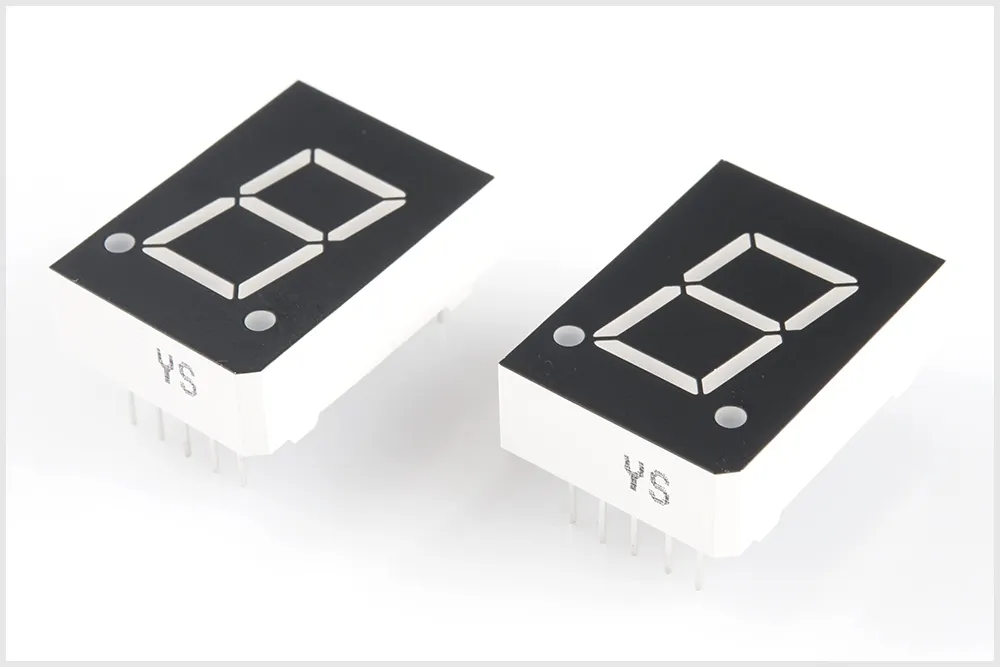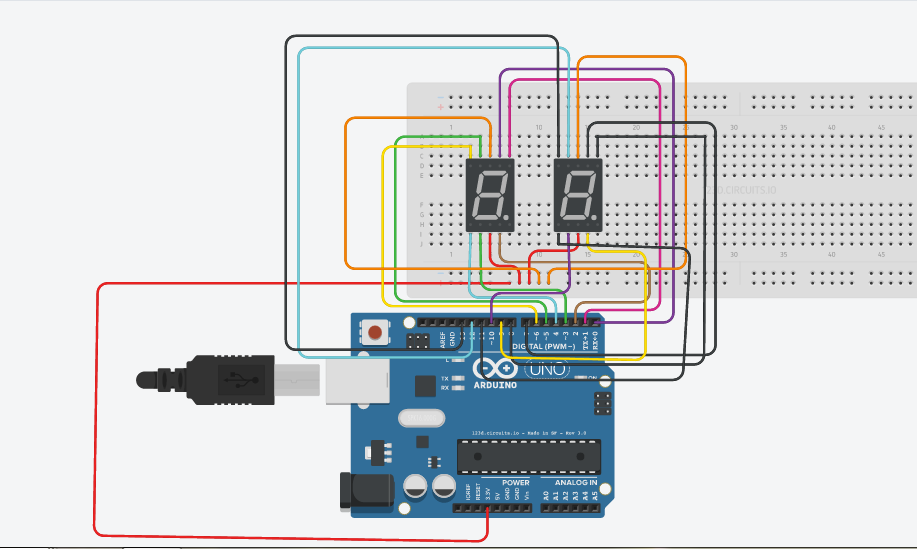00 to 99 on Seven-Segment Displays

Using two Seven-Segment Displays (SSD) simultaneously on an Arduino Uno Board to display numbers from 00 to 99, without charlieplexing.

• 78,649 views
• 35 respects

Components and supplies

This is a basic project for all the beginners.

We are not using the charlieplexing method here.

Here we will display the numbers from 00 to 99 on two Seven-Segment Displays(SSD) which we will use simultaneously.

I have made this project using an Arduino Uno Board.

We need the following to make this:

• Arduino Uno - 1
• Programmer Cable - 1
• Seven-Segment Display - 2
• Male-Male Jumper Wires

Which Seven-Segment Display is used here?

There are two types- Common Anode and Common Cathode.

Here I have used two Common Anode SSDs.

If you are using Common Cathode ones, You just need to convert the 0's to 1's and the vice-versa.

We will be using the pins from 0 to 13 on the Arduino Board, not connecting the dot as we do not require it.

SSD(1) here refers to the SSD which displays the tens digit.

SSD(2) here refers to the SSD which displays the ones digit.

Connecting the Arduino pins to SSD pins:

Attach the pins 3 and 8 on both the SSD's to 3.3V.(Use resistors)

Since this is for Common Anode SSD, the LED glows when it is LOW/0.

If you are using a Common Cathode SSD, attach pins 3 and 8 to ground and then the LEDs glow when attached to HIGH/1.

Do not let 5 V pass through any of your LEDs, You must use resistors.

Result: Numbers from 00 to 99 are displayed.

Here is the video:

Code

Here is the code for this basic project.C/C++
This is the code I used.
//SSD is Seven-Segment Display

void setup()
{
for (int i = 0; i <= 13; i++)
pinMode(i, OUTPUT); //Set all pins from 0 to 13 as OUTPUT
}
//The line below is the array containing all the binary numbers for the digits on a SSD from 0 to 9
const int number = {0b1000000, 0b1111001, 0b0100100, 0b0110000, 0b0011001, 0b0010010, 0b0000010, 0b1111000, 0b0000000, 0b0010000};

void loop()
{
for (int tens = 0; tens < 10; tens++)

{
display_tens(tens);
}
}

void display_tens(const int tens)
{
int pin1, a, ones;
//pin1 is just used to deal with pins of the 1st SSD which desplays the tens digit

for (pin1 = 0, a = 0; pin1 < 7; pin1++, a++)
{
}
for (ones = 0; ones < 10; ones++)
{
display_ones(ones);
delay(300);
//I have given a delay of 300 milliseconds. You can put your own Time!!
}
}

void display_ones(const int x)
{ int pin2, b;
//pin2 is just used to deal with pins of the 2nd SSD which desplays the ones digit

for (pin2 = 7, b = 0; pin2 <= 13; pin2++, b++)
{

}

}

Schematics

Here is how I connected the jumper wires on the board.The resistors are not shown here.• 4,790 views
• 8 respects

Ultimate Beginner’s Guide to Run TFT LCD Displays by Arduino

• 131,090 views
• 99 respects

• 25,185 views
• 15 respects

• 10,877 views
• 1 comment
• 14 respects

How to make Arduino Piano keyboard

Project tutorial by Sharifdeen Ashshak

• 6,280 views